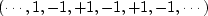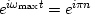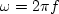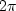Next: Fourier sum Up: FOURIER SUMS Previous: Superposition of sinusoids

## Sampled time and Nyquist frequency

In the world of computers, time is generally mapped into integers too, say.This is called discretizing'' or sampling.'' The highest possible frequency expressible on a mesh is,which is the same as.Setting,we see that the maximum frequency is(18)
Time is commonly given in either seconds or sample units, which are the same when.In applications, frequency is usually expressed in cycles per second, which is the same as Hertz, abbreviated Hz. In computer work, frequency is usually specified in cycles per sample. In theoretical work, frequency is usually expressed in radians where the relation between radians and cycles is.We use radians because, otherwise, equations are filled with's. When time is given in sample units, the maximum frequency has a name: it is the Nyquist frequency,'' which isradians or 1/2 cycle per sample.Next: Fourier sum Up: FOURIER SUMS Previous: Superposition of sinusoids
Stanford Exploration Project
10/21/1998